NEET  >  NEET Previous Year Questions (2014-22): Electric Charges & Fields

# NEET Previous Year Questions (2014-22): Electric Charges & Fields - Notes | Study Physics Class 12 - NEET

 1 Crore+ students have signed up on EduRev. Have you?

Q.1. Two point charges –q and +q are placed at a distance of L, as shown in the figure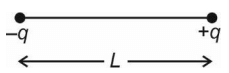The magnitude of electric field intensity at a distance R(R >>L) varies as:              (2022)
(1) 1/R3

(2) 1/R4
(3)1/R6
(4) 1/R2

For R >>L, arrangement is an electric dipole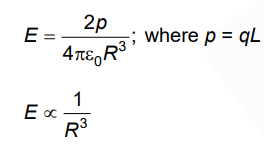Q.2. Polar molecules are the molecules:      (2021)
A: acquire a dipole moment only when magnetic field is absent.
B: having a permanent electric dipole moment.
C: having zero dipole moment.
D: acquire a dipole moment only in the presence of electric field due to displacement of charges.
Ans:
B
Polar molecules have centres of positive and negative charges separated by some distance, so they have permanent dipole moment.

Q.3. A dipole is placed in an electric field as shown. In which direction will it move?        (2021)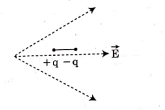A: towards the left as its potential energy will decrease.
B: towards the right as its potential energy will increase.
C: towards the left as its potential energy will increase.
D: towards the right as its potential energy will decrease.
Ans:
D
E1| > |E2| as field lines are closer at charge +q,  so net force on the dipole acts towards right side.
A system always moves to decrease it's potential energy.Q.4. A short electric dipole has a dipole moment of 16×10-9 C m. The electric potential due to the dipole at a point at a distance of 0.6 m from the centre of the dipole, situated on a line making an angle of 60° with the dipole axis is:      (2020)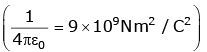A:  400 V
B: Zero
C: 50 V
D: 200 V
Ans:
D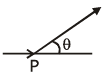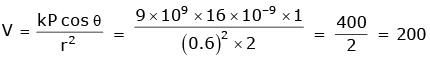Q.5. A hollow metal sphere of radius R is uniformly charged. The electric field due to the sphere at a distance r from the centre.     (2019)
A: Increases as r increases for both r < R and r > R
B: Zero as r increases for r < R, decreases as r increases for r > R
C: Zero as r increases for r < R, increases as r increases for r > R
D:
Decreases as r increases for both r < R and r > R
B
Solution: Charge Q will be distributed over the surface of hollow metal sphere.
(i) For r < R (inside)
By Gauss law,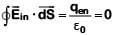⇒ Ein = 0  (∵ qen = 0)
(ii) For r > R (outside)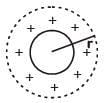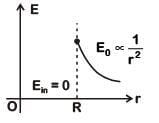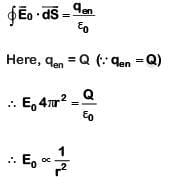Q.6. Two parallel infinite line charges with linear charge densities +λ C/m and λl C/m are placed at a distance of 2R in free space. What is the electric field mid-way between the two line charges?     (2019)
A: Zero
B: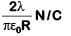C: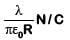D: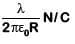C
Solution: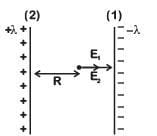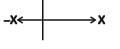Electric field due to line charge (1)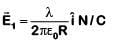Electric field due to line charge (2)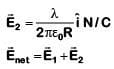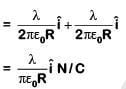Q.7. Two point charges A and B, having charges +Q and –Q respectively, are placed at certain distance apart and force acting between them is F. If 25% charge of A is transferred to B, then force between the charges becomes :     (2019)
A: F
B: 9F/16
C: 16F/9
D: 4F/3
B
Solution: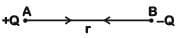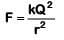If 25% of charge of A transferred to B then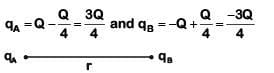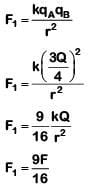Q.8. An electron falls from rest through a vertical distance h in a uniform and vertically upward directed electric field E. The direction of electrical field is now reversed, keeping its magnitude the same. A proton is allowed to fall from rest in through the same vertical distance h. The time fall of the electron, in comparison to the time fall of the proton is :-      (2018)
A: smaller
B: 5 times greater
C: 10 times greater
D: equal
A
Solution: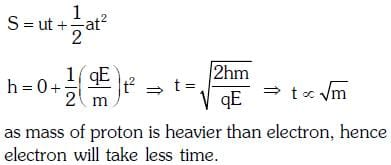Q.9. A toy car with charge q moves on a frictionless horizontal plane surface under the influence of a uniform electric field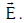Due to the force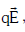its velocity increases from 0 to 6 m/s in one second duration. At that instant the direction of the field is reversed. The car continues to move for two more seconds under the influence of this field. The average velocity and the average speed of the toy car between 0 to 3 seconds are respectively :-    (2018)
A: 2 m/s, 4 m/s
B: 1 m/s, 3 m/s
C: 1 m/s, 3.5 m/s
D: 1.5 m/s, 3 m/s
B
Solution:
0 < t < 1s : velocity increases from 0 to 6 m/s
1 < t < 2s : velocity decreases from 6 to 0 m/s
but car continues to move forward
2 < t < 3s : since field strength is same ⇒ same acceleration
∴ car's velocity increases
from 0 to –6 m/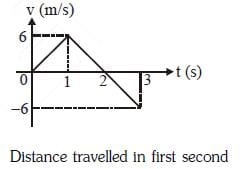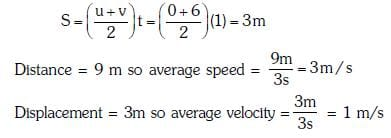Q.10. Two identical charged spheres suspended from a common point by two mass less strings of lengths ℓ are initially at a distance d(d << ℓ) a part because of their mutual repulsion. The charges begin to leak from both the spheres at a constant rate. As a result, the spheres approach each other with a velocity v. Then v varies as a function of the distance x between the spheres, as :    (2016)
A: v ∝ x-1
B: v ∝ x1/2
C: v ∝ x
D: v ∝ x-1/2
D
Solution: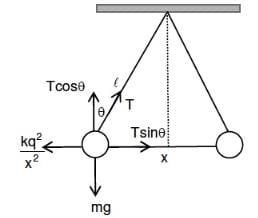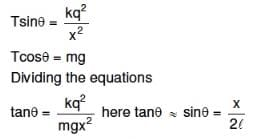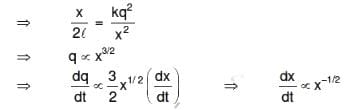Q.11. A square loop ABCD carrying a current i, is placed near and coplanar with a long straight conductor XY carrying a current I, the net force on the loop will be :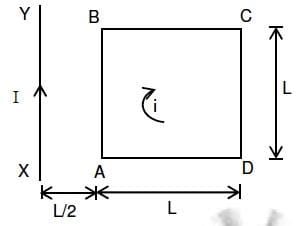A: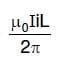B: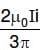C: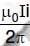D: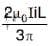Solution: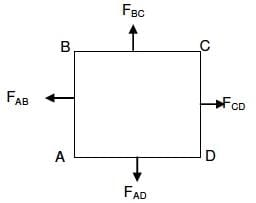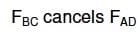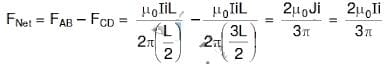Q.12. The electric field in a certain region is acting radially outward and is given by E = Ar. A charge contained in a sphere of radius = a centred at the origin of the field, will be given by :     (2015)
A: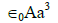B: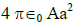C: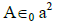D: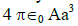D
Solution: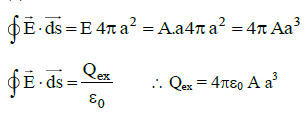Q.13. A wire carrying current I has the shape as shown in the adjoining figure. Linear parts of the wire are very long and parallel to X  -axis while semicircular portion of radius R is lying in a Y-Z plane. The magnetic field at point O is.     (2015)
A: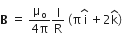B: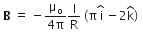C: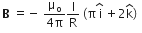D: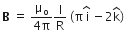C
Solution:
The magnetic fiedl in the different regions is given by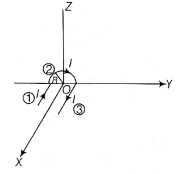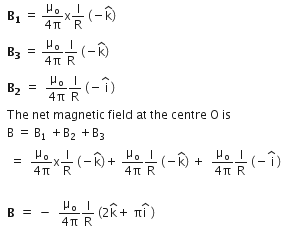Q.14. An electron moving in a circular orbit of radius r makes n rotations per second. The magnetic field produced at the centre has magnitude :     (2015)
A: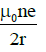B: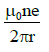C: zero
D: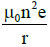Solution: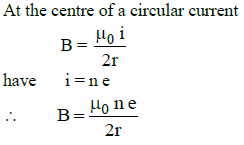Q.15. Following figures show the arrangement of bar magnets in different configurations. Each magnet has magnetic dipole moment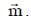Which configuration has highest net magnetic dipole moment?     (2014)
A: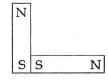B: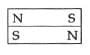C: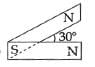D: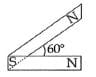C
Solution:
The direction of magnetic moment is from S to N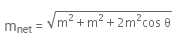Net magnetic moment will be maximum if cos θ is maximum.
cos θ will be maximum when θ will be minimum. So, at θ = 30º mnet will be maximum.
The direction of magnetic moment is from S to N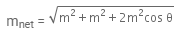Net magnetic moment will be maximum if cos θ is maximum.
cos θ will be maximum when θ will be minimum. So, at θ = 30º mnet will be maximum.

The document NEET Previous Year Questions (2014-22): Electric Charges & Fields - Notes | Study Physics Class 12 - NEET is a part of the NEET Course Physics Class 12.
All you need of NEET at this link: NEET

## Physics Class 12

157 videos|452 docs|213 tests
 Use Code STAYHOME200 and get INR 200 additional OFF

## Physics Class 12

157 videos|452 docs|213 tests

### How to Prepare for NEET

Read our guide to prepare for NEET which is created by Toppers & the best Teachers

Track your progress, build streaks, highlight & save important lessons and more!

,

,

,

,

,

,

,

,

,

,

,

,

,

,

,

,

,

,

,

,

,

;